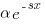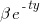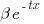## Moments Of Pathological Behaviour – a.k.

Last time we took a look at basis function interpolation with which we approximate functions from their values at given sets of arguments, known as nodes, using weighted sums of distinct functions, known as basis functions. We began by constructing approximations using polynomials before moving on to using bell shaped curves, such as the normal probability density function, centred at the nodes. The latter are particularly useful for approximating multi-dimensional functions, as we saw by using multivariate normal PDFs.
An easy way to create rotationally symmetric functions, known as radial basis functions, is to apply univariate functions that are symmetric about zero to the distance between the interpolation's argument and their associated nodes. PDFs are a rich source of such functions and, in fact, the second bell shaped curve that we considered is related to that of the Cauchy distribution, which has some rather interesting properties.

## Calculating statement execution likelihood

In the following code, how often will the variable `b` be incremented, compared to `a`?

If we assume that the variables `x` and `y` have values drawn from the same distribution, then the condition `(x < y)` will be true 50% of the time (ignoring the situation where both values are equal), i.e., `b` will be incremented half as often as `a`.

```a++;
if (x < y)
{
b++;
if (x < z)
{
c++;
}
}
```

If the value of `z` is drawn from the same distribution as `x` and `y`, how often will `c `be incremented compared to `a`?

The test `(x < y)` reduces the possible values that `x` can take, which means that in the comparison `(x < z)`, the value of `x` is no longer drawn from the same distribution as `z`.

Since we are assuming that `z` and `y` are drawn from the same distribution, there is a 50% chance that `(z < y)`.

If we assume that `(z < y)`, then the values of `x` and `z` are drawn from the same distribution, and in this case there is a 50% change that `(x < z)` is true.

Combining these two cases, we deduce that, given the statement `a++;` is executed, there is a 25% probability that the statement `c++;` is executed.

If the condition `(x < z)` is replaced by `(x > z)`, the expected probability remains unchanged.

If the values of `x`, `y`, and `z` are not drawn from the same distribution, things get complicated.

Let's assume that the probability of particular values of `x` and `y` occurring areand, respectively. The constantsandare needed to ensure that both probabilities sum to one; the exponentsandcontrol the distribution of values. What is the probability that `(x < y)` is true?

Probability theory tells us that, where:is the probability distribution function for(in this case:), andthe the cumulative probability distribution for(in this case:).

Doing the maths gives the probability of `(x < y)` being true as:.

The `(x < z)` case can be similarly derived, and combining everything is just a matter of getting the algebra right; it is left as an exercise to the reader :-)

## Archimedean Crew – a.k.

We have recently seen how we can define dependencies between random variables with Archimedean copulas which calculate the probability that they each fall below given values by applying a generator function φ to the results of their cumulative distribution functions, or CDFs, for those values, and applying its inverse to their sum.
Like all copulas they are effectively the CDFs of vector valued random variables whose elements are uniformly distributed when considered independently. Whilst those Archimedean CDFs were relatively trivial to implement, we found that their probability density functions, or PDFs, were somewhat more difficult and that the random variables themselves required some not at all obvious mathematical manipulation to get right.
Having done all the hard work implementing the `ak.archimedeanCopula`, `ak.archimedeanCopulaDensity` and `ak.archimedeanCopulaRnd` functions we shall now use them to implement some specific families of Archimedean copulas.

## Archimedean Review – a.k.

In the last couple of posts we've been taking a look at Archimedean copulas which define the dependency between the elements of vector values of a multivariate random variable by applying a generator function φ to the values of the cumulative distribution functions, or CDFs, of their distributions when considered independently, known as their marginal distributions, and applying the inverse of the generator to the sum of the results to yield the value of the multivariate CDF.
We have seen that the densities of Archimedean copulas are rather trickier to calculate and that making random observations of them is trickier still. Last time we found an algorithm for the latter, albeit with an implementation that had troubling performance and numerical stability issues, and in this post we shall add an improved version to the `ak` library that addresses those issues.

## Archimedean View – a.k.

Last time we took a look at how we could define copulas to represent the dependency between random variables by summing the results of a generator function φ applied to the results of their cumulative distribution functions, or CDFs, and then applying the inverse of that function φ-1 to that sum.
These are known as Archimedean copulas and are valid whenever φ is strictly decreasing over the interval [0,1], equal to zero when its argument equals one and have nth derivatives that are non-negative over that interval when n is even and non-positive when it is odd, for n up to the number of random variables.
Whilst such copulas are relatively easy to implement we saw that their densities are a rather trickier job, in contrast to Gaussian copulas where the reverse is true. In this post we shall see how to draw random vectors from Archimedean copulas which is also much more difficult than doing so from Gaussian copulas.

## Archimedean Skew – a.k.

About a year and a half ago we saw how we could use Gaussian copulas to define dependencies between the elements of a vector valued multivariate random variable whose elements, when considered in isolation, were governed by arbitrary cumulative distribution functions, known as marginals. Whilst Gaussian copulas are quite flexible, they can't represent every possible dependency between those elements and in this post we shall take a look at some others defined by the Archimedean family of copulas.

## A Measure Of Borel Weight – a.k.

In the last few posts we have implemented a type to represent Borel sets of the real numbers, which are the subsets of them that can be created with countable unions of intervals with closed or open lower and upper bounds. Whilst I would argue that doing so was a worthwhile exercise in its own right, you may be forgiven for wondering what Borel sets are actually for and so in this post I shall try to justify the effort that we have spent on them.

## A Borel Universe – a.k.

Last time we took a look at Borel sets of real numbers, which are subsets of the real numbers that can be represented as unions of countable sets of intervals Ii. We got as far as implementing the `ak.borelInterval` type to represent an interval as a pair of `ak.borelBound` objects holding its lower and upper bounds.
With these in place we're ready to implement a type to represent Borel sets and we shall do exactly that in this post.

## A Decent Borel Code – a.k.

A few posts ago we took a look at how we might implement various operations on sets represented as sorted arrays, such as the union, being the set of every element that is in either of two sets, and the intersection, being the set of every element that is in both of them, which we implemented with `ak.setUnion` and `ak.setIntersection` respectively.
Such arrays are necessarily both finite and discrete and so cannot represent continuous subsets of the real numbers such as intervals, which contain every real number within a given range. Of particular interest are unions of countable sets of intervals Ii, known as Borel sets, and so it's worth adding a type to the `ak` library to represent them.